# Integral logarithm

The special function defined, for positive real,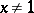, by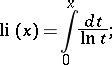forthe integrand has atan infinite discontinuity and the integral logarithm is taken to be the principal value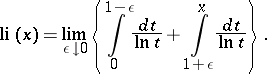The graph of the integral logarithm is given in the article Integral exponential function. Forsmall: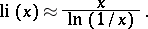The integral logarithm has for positive realthe series representationwhereis the Euler constant. As a function of the complex variable,is a single-valued analytic function in the complex-plane with slits along the real axis fromto 0 and from 1 to(the imaginary part of the logarithms is taken within the limitsand). The behaviour of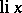along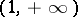is described by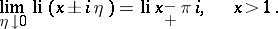The integral logarithm is related to the integral exponential functionbyFor real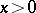one sometimes uses the notation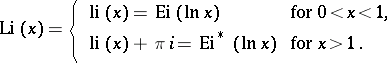For references, see Integral cosine.

The function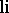is better known as the logarithmic integral. It can, of course, be defined by the integral (as above) for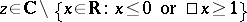.
The series representation for positive,, is then also said to define the modified logarithmic integral, and is the boundary value of,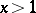,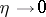. For realthe value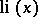is a good approximation of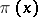, the number of primes smaller than(see de la Vallée-Poussin theorem; Distribution of prime numbers; Prime number).